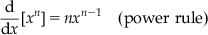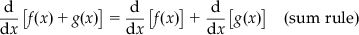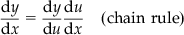Table 1.1

Sample Derivative Rules (c and n are Arbitrary Constrants)

 1. $\frac{\mathrm{d}}{\mathrm{d}x}\left[{x}^{n}\right]=n{x}^{n-1}\left(\text{power rule}\right)$2. $\frac{\mathrm{d}}{\mathrm{d}x}\left[f\left(x\right)+g\left(x\right)\right]=\frac{\mathrm{d}}{\mathrm{d}x}\left[f\left(x\right)\right]+\frac{\mathrm{d}}{\mathrm{d}x}\left[g\left(x\right)\right]\left(\text{sum rule}\right)$3. $\frac{\mathrm{d}y}{\mathrm{d}x}=\frac{\mathrm{d}y}{\mathrm{d}u}\frac{\mathrm{d}u}{\mathrm{d}x}\left(\text{chain rule}\right)$4. $\frac{\mathrm{d}}{\mathrm{d}x}\left[f\left(x\right)g\left(x\right)\right]=f\left(x\right)\frac{\mathrm{d}}{\mathrm{d}x}\left[g\left(x\right)\right]+g\left(x\right)\frac{\mathrm{d}}{\mathrm{d}x}\left[f\left(x\right)\right]\left(\text{product rule}\right)$5. $\frac{\mathrm{d}}{\mathrm{d}x}\left[\frac{f\left(x\right)}{g\left(x\right)}\right]=\frac{g\left(x\right)\frac{\mathrm{d}}{\mathrm{d}x}\left[f\left(x\right)\right]-f\left(x\right)\frac{\mathrm{d}}{\mathrm{d}x}\left[g\left(x\right)\right]}{{\left[g\left(x\right)\right]}^{2}}\left(\text{quotient rule}\right)$ 6. $\frac{\mathrm{d}}{\mathrm{d}x}\left[cf\left(x\right)=c\frac{\mathrm{d}}{\mathrm{d}x}\left[f\left(x\right)\right]\left(\text{constant multiple ...}$

Get Risk Neutral Pricing and Financial Mathematics: A Primer now with the O’Reilly learning platform.

O’Reilly members experience books, live events, courses curated by job role, and more from O’Reilly and nearly 200 top publishers.